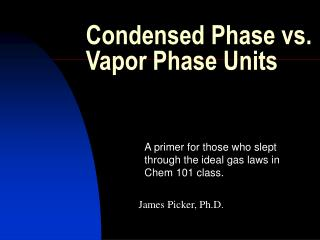Download PresentationCondensed Phase vs. Vapor Phase Units

# Condensed Phase vs. Vapor Phase Units - PowerPoint PPT PresentationDownload Presentation## Condensed Phase vs. Vapor Phase Units

- - - - - - - - - - - - - - - - - - - - - - - - - - - E N D - - - - - - - - - - - - - - - - - - - - - - - - - - -
##### Presentation Transcript

1. Condensed Phase vs. Vapor Phase Units A primer for those who slept through the ideal gas laws in Chem 101 class. James Picker, Ph.D.

2. Condensed Phase:Solids • ug/g defined as ppm: because microX (one millionth of X) per X defined as ppm • ug/kg defined as ppb : because microX (one millionth of X) per 1000X defined as ppb OR • nano X ( one billionth of X) per X defined as ppb

3. Condensed Phase:Liquids • ug/mL defined as ppm: because microX (one millionth of X) per X defined as ppm • ug/L defined as ppb : because microX (one millionth of X) per 1000X defined as ppb • BECAUSE • One mL of water weighs approx 1 gram • One L of water weighs approx 1 kg

4. 1 pint in a 25 meter pool Half a drop in a bucket (5 gallon) A penny in \$10,000 A minute in two years Examples of ppm

5. 10 drops in a 25 meter pool An amount too small to see in a bucket (5 gallon) A penny in \$10,000,000 A second in 32 years Examples of ppb

6. VAPOR PHASE • Definition of ppm(v/v) = microX/X • In gas phase that is: • =uL(compound)/Liter(total vapor) • Next Determine weight of 1 uL • From PV=nRT rearange to • n=PV/RT where n = moles • nM=PVM/RT where M= mole weight • nM=Grams= • (1L)(1x10-6)(M)/(.0821)(298K)

7. VAPOR PHASE • Grams=(M/24.5) X 10-6 • For Benzene: 3.2 ug/L = 1ppmv • and 3.2 ug/m3= 1ppbv • For TCE: 5.4 ug/L = 1ppmv • and 5.4 ug/m3= 1ppbv • Note: m3= 1000L